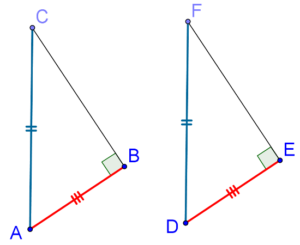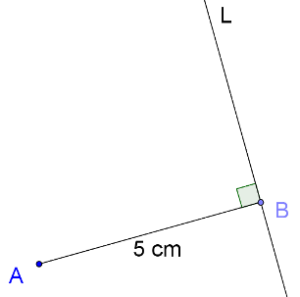# The RHS Criterion

Go back to  'Triangles'

We have seen that there is no such thing as an SSA criterion. That is, if two sides and a non-included angle in one triangle are equal to two sides and a non-included angle in another triangle, we cannot say that the two triangles are congruent. Let us revisit that statement once again. What if the non-included angle happens to be a right angle?

Consider two triangles, ∆ABC and ∆DEF, where ∠B = ∠E = 90°, the hypotenuses AC and DF are equal, and one pair of legs is equal, say, AB and DE, as shown below:Note that the SAS criterion for congruence does not apply, since the right angle is not the included angle between the two pairs of equal sides. Can we still say that the two triangles are congruent?

Yes, we can. Before going on to the proof, you must think about this intuitively. The best way is through an exercise. Suppose that you are asked to construct a right angled triangle whose hypotenuse is 10 cm, and one of whose legs is 5 cm. How many such different triangles can you construct?

You will find that you can construct only one such triangle. Here is a step-by-step breakdown of the construction process:

1. Draw a line segment AB of length 5 cm.2. Through B, construct a line L perpendicular to AB:3. Measure a length of 10 cm between the tip of your compass and the pencil’s tip.

4. Keeping the tip of your compass at A, mark off a point C on L such that AC = 10cm. There you have it, the right triangle you were asked to construct:You may say that there can be two positions for C, one above and one below AB. However, a little thinking will show that the two triangles you thus obtain will actually be the same (as in, congruent), so you end up with only one distinct triangle.

Thus, you can draw only one right angled triangle with given lengths of the hypotenuse and a leg. In other words, if the hypotenuses and one pair of legs of two right angled triangles are (respectively) equal, then they must be congruent.

grade 9 | Questions Set 1